## 3.4Equation discretisation

Equation discretisation converts partial diﬀerential equations for continuous ﬁelds, e.g. pressure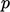, into sets of linear equations for discrete ﬁelds.

The values of the principal ﬁelds, e.g., are associated with cells. A ﬁeld is then represented by an array of values,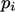, for cell indices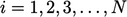.is the total number of cells.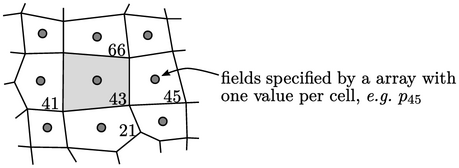Equation discretisation creates a linear equation for each cell. For cell 43 above, the equation might have the following form: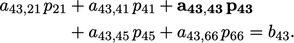where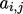and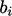are coeﬃcients corresponding to cell indices,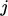(diagonal coeﬃcient in bold). The set of linear equations for all cells can be written as a matrix equation of the form: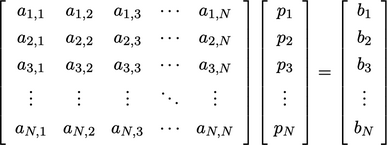(3.1)
The matrix contains of a set of coeﬃcients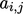where each rowcorresponds to the linear equation for the cell with index. Each row of coeﬃcients are non-zero only for the respective cell (diagonal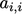) and near-neighbours. All other coeﬃcients are zero, making the matrix extremely sparse.

The matrix equation can be represented as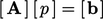, where:are the matrix coeﬃcients;are the source coeﬃcients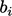; and,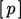is the discretised pressure ﬁeld. It may also be illustrated as follows, where ‘’ indicates non-zero coeﬃcients.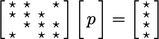### Segregated solution

A CFD simulation generally solves a set of physical equations, e.g. for mass, momentum and energy conservation. The ﬁnite volume method traditionally discretises each physical equation separately to form individual matrix equations for single solution variables, e.g.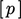,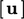,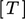, rather than creating a single matrix equation that represents all the physical equations.

The segregated matrix equations are solved one variable at a time, e.g. solving for,andin separate steps. Where the solution variable is a vector or tensor, e.g.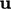, it is decoupled into individual matrix equations for each component, e.g.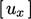,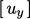,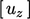.

The matrix equations are solved in an iterative sequence, in which the equation for one variable, e.g.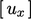, incorporates current values of other variables, e.g.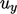,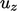and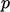, into the source vector, as shown below.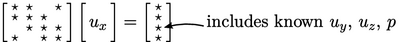Notes on CFD: General Principles - 3.4 Equation discretisation